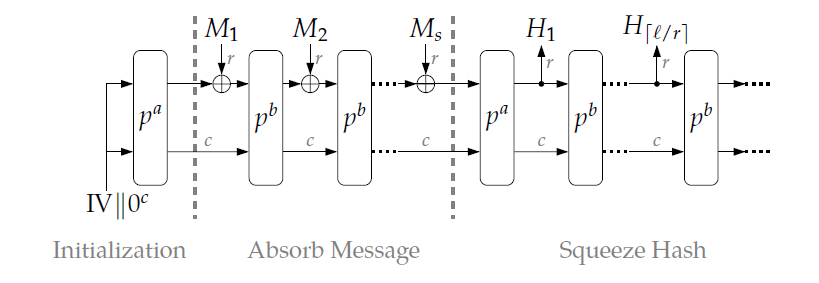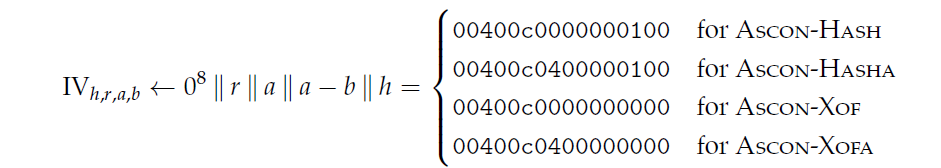• 累计撰写 74 篇文章
• 累计创建 79 个标签
• 累计收到 31 条评论

### 目 录CONTENT# Ascon-Hash过程详解2023-07-27 / 0 评论 / 3 点赞 / 139 阅读 / 1,654 字

# 海绵结构图# 参数

• 256：表示输出为256比特的哈希值
• 64：表示对输入数据分组，每组为64比特
• 12、12：表示 $p^a、p^b$中的 $a=12、b=12$ ，也就是均处理12次

# 过程详解

## 初始化Initialization

• 状态S的构成：状态S是320bits, $S=p^a (Ⅳ_{h,r,a,b} \| 0^{256})$
• 上述$Ⅳ_{h,r,a,b}$请看下图，我们选用的是第一个，$h=256,r=64,a=12,b=12$
• 由于任何使用Ascon-Hash的状态S初试都是一样的，这里直接给出状态S的结果可以用于检验（下划线只是为了分隔）## 吸收数据Absorb Message

同理，对于输入数据M需要先填充与分组，请看填充Padding，与明文一样的填充法。在后面填充一个比特的1和若干比特的0直到输入数据M的长度为64的倍数。$M \rightarrow M\|1\|0^{r-1-(|M| \quad mod \quad r)}$，填充完之后进行分组，每组64比特，共s组。

• 对于前s-1组，即 $i=1 \rightarrow s-1$$S=p^b((S_r \oplus M_i) \| S_c)$，其中b=12，在前面说过的，这个与Ascon加密中b=6有所不同。
• 将状态S的前64位与输入数据的第i组进行异或，然后再执行一遍 $p^b$过程。
• 对于第s组，即$i=s$$S=(S_r \oplus M_s) \| S_c$
• 将状态S的前64位与输入数据的第s组进行异或，不需要再执行 $p^b$过程。

## 压缩哈希 Squeeze Hash

最后的哈希输出由上述的状态S获得，与输入数据已经无关了。记哈希值为H

• 首先，先执行一遍 $S=p^a(S)$
• 之后，再生成哈希值H
• 对于$1\leq i \leq t=\lceil \frac{h}{r} \rceil$，其中h=256，r=64均在前面提到过，至于为什么不直接写t=4，是因为ASCON的有些哈希算法规定输出长度不是64的整数倍。
• $H_i = S_r$
• $S=p^b(S)$
• 由上述我们可以得到 $H_1,H_2,...,H_t$，每个都是64位，由于有些哈希值不是64的整数倍，因此需要对$H_t$进行切割
• $H_t' = \lfloor H_t\rfloor_{h\ mod\ r}$，只截取$H_t$的前 h 取余 r 比特
• 然后再拼接：$H=H_1 \| H_2 \| H_3 \|...\|H_t'$，得到h比特的哈希值

# 代码详解

## 定义数据类型

using ascon_hash = std::vector<ascon_64>;  // 哈希值的数据类型


## 定义常量

static const permutations_type hash_a = 12;
static const permutations_type hash_b = 12;
static const int hash_length = 256;


## 哈希过程

// 计算哈希
void Asconv12::hash(const ascon_data& message, ascon_hash& hashVal) {
// 哈希过程初始化
ascon_state S;
S.resize(5);
S = 0x00400c0000000100;
S = 0;
S = 0;
S = 0;
S = 0;
Asconv12::permutations(Asconv12::hash_a, S);
if (S == 0xee9398aadb67f03d and S == 0x8bb21831c60f1002 and S == 0xb48a92db98d5da62 and S == 0x43189921b8f8e3e8 and S == 0x348fa5c9d525e140) {
// std::cout << "初始化成功" << std::endl;
}

// 哈希过程吸收数据
if(message.empty()) M = {0x8000000000000000};  // 哈希过程中，是必须要填充的，哪怕为空
for (int i = 0; i < M.size() - 1; i++) {
S ^= M[i];
Asconv12::permutations(Asconv12::hash_b, S);
}
S ^= M.back();

// 哈希过程压缩数据
if (!hashVal.empty()) hashVal.clear();
int size_of_out = Asconv12::hash_length % Asconv12::r == 0 ? Asconv12::hash_length / Asconv12::r : Asconv12::hash_length / Asconv12::r + 1;
hashVal.resize(size_of_out);

Asconv12::permutations(Asconv12::hash_a, S);
for (int i = 0; i < hashVal.size(); ++i) {
hashVal[i] = S;
Asconv12::permutations(Asconv12::hash_b, S);
}
ascon_64 tmp = 0xffffffffffffffff;
for (int i = 0; i < (Asconv12::hash_length % Asconv12::r); ++i) {
tmp <<= 1;
}
hashVal[hashVal.size() - 1] &= tmp;
}


### 初始化

// 哈希过程初始化
ascon_state S;
S.resize(5);

S = 0x00400c0000000100; // Ⅳ的值
S = 0; S = 0; S = 0; S = 0;  // 这里只是说明后面填充的0 S=Ⅳ||0^256
Asconv12::permutations(Asconv12::hash_a, S);  // p^a过程

// 下面是为了验证计算是否正确的，前面我们直接给出了ASCON-HASH的初始化之后的状态S值
S == 0x8bb21831c60f1002 and
S == 0xb48a92db98d5da62 and
S == 0x43189921b8f8e3e8 and
S == 0x348fa5c9d525e140) {
// std::cout << "初始化成功" << std::endl;
}


## 吸收数据

ascon_padding M;
for (int i = 0; i < M.size() - 1; i++) {  // 前 s-1 组数据
S ^= M[i];
Asconv12::permutations(Asconv12::hash_b, S);
}

S ^= M.back();  // 第S组数据，单独处理


## 压缩数据

Asconv12::permutations(Asconv12::hash_a, S); // 首先进行p^a过程
for (int i = 0; i < hashVal.size(); ++i) {  // 处理1-t的数据
hashVal[i] = S;
Asconv12::permutations(Asconv12::hash_b, S);
}

// 对于 Ht 需要单独处理，也就是截取Ht为Ht'
ascon_64 tmp = 0xffffffffffffffff;
for (int i = 0; i < (Asconv12::hash_length % Asconv12::r); ++i) {
tmp <<= 1;
}
hashVal[hashVal.size() - 1] &= tmp;

3
1.2.• 3
1.2.